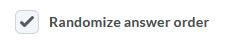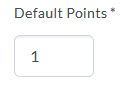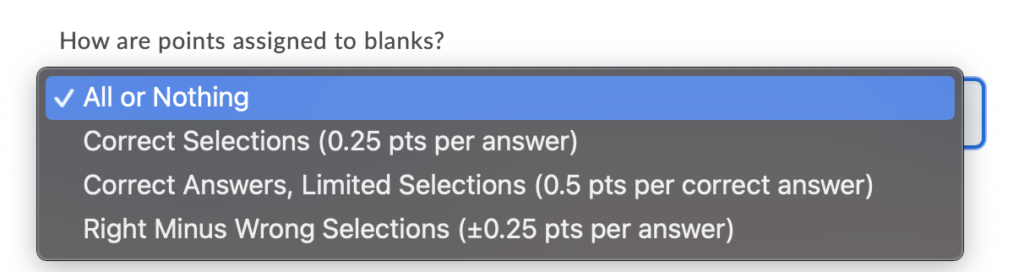# Create Multi-Select Questions in the Question Library

Watch how to create a multi-select question

Multi-select (M-S) questions require respondents to identify one or more correct answers in a list of possible answers. Unlike multiple choice (MC) questions, multi-select questions enable you to choose a grading format and allow students to select more than one answer.

1. On the nav bar, select the Activities menu, then click either Quizzes, Surveys, or Self Assessments. In the example below, we selected Quizzes.2. From the tool navigation, click Question Library.3. Select New.4. Select the question type: Multi-Select Question (M-S).When instructors initially launch the Question Editor to create a multi-select question, the interface displays the two main components of a multi-select question: the question and potential answers.
5. In the Question Text field, enter your multi-select question. Selecting a field displays a pop-up toolbar for formatting the text and adding images, links, and graphical equations. As each field is completed, the preview pane displays how the question and answers appear to students.6. In the Answers area, enter the answers for the multi select question. Check the box next to each correct answer. Selecting a field displays a pop-up toolbar for formatting the text and adding images, links, and graphical equations. As each field is completed, the preview pane displays how the question and answers appear to students.8. If you want to allow each student to view the answer options in a random order, select the Randomize Answer Order checkbox.9. Set the number of points for the multi select question.There are four possible grading options for multi-select questions:

• All or nothing – Students earn full points only when all correct answers are selected and all incorrect answers are left blank. Students cannot earn partial points.
• Correct Selections – Points are evenly distributed across all answers. Students earn partial points for each answer correctly selected and left blank.
• Correct answers, Limited selections – Points are evenly distributed across correct answers only. The number of selections allowed is limited to the number of correct answers. Students earn partial points for each correct answer selected.
• Right minus wrong –  Points are evenly distributed across all answers. Learners earn partial points for each answer correctly selected and left blank. Learners lose points for answers incorrectly selected or left blank. Learners cannot receive less than 0 points.
• To calculate how much each answer is worth, the system takes the total number of points assigned to the question and divides it by the total number of answer choices.
• For example, if a question is worth 10 points total and has 5 answer choices, each choice is worth 2 points (10/5=2). Correct answers are worth +2 points each and incorrect answers are worth -2 points each. If the student selects 3 correct answers and 2 incorrect answers, they would receive 2 points for each correct answer and lose 2 points for each incorrect answer, resulting in a total score of 2 points for the question [3(2)+2(-2)=2].

11. From the Options area, optionally, specify the following: